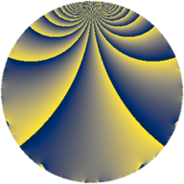# Properties

 Label 637.2.cLevel $637$ Weight $2$ Character orbit 637.c Rep. character $\chi_{637}(246,\cdot)$ Character field $\Q$ Dimension $44$ Newform subspaces $7$ Sturm bound $130$ Trace bound $3$

# Related objects

## Defining parameters

 Level: $$N$$ $$=$$ $$637 = 7^{2} \cdot 13$$ Weight: $$k$$ $$=$$ $$2$$ Character orbit: $$[\chi]$$ $$=$$ 637.c (of order $$2$$ and degree $$1$$) Character conductor: $$\operatorname{cond}(\chi)$$ $$=$$ $$13$$ Character field: $$\Q$$ Newform subspaces: $$7$$ Sturm bound: $$130$$ Trace bound: $$3$$ Distinguishing $$T_p$$: $$2$$, $$3$$

## Dimensions

The following table gives the dimensions of various subspaces of $$M_{2}(637, [\chi])$$.

Total New Old
Modular forms 72 54 18
Cusp forms 56 44 12
Eisenstein series 16 10 6

## Trace form

 $$44 q - 40 q^{4} + 36 q^{9} + O(q^{10})$$ $$44 q - 40 q^{4} + 36 q^{9} + 20 q^{12} - 8 q^{13} + 24 q^{16} + 8 q^{17} - 36 q^{22} - 16 q^{23} - 36 q^{25} - 12 q^{26} + 12 q^{27} + 8 q^{29} - 44 q^{30} - 20 q^{36} + 4 q^{38} + 4 q^{39} + 20 q^{40} - 24 q^{43} - 8 q^{48} - 48 q^{51} + 20 q^{52} + 40 q^{53} - 12 q^{55} - 28 q^{61} - 16 q^{62} - 36 q^{64} + 16 q^{65} - 28 q^{66} - 20 q^{68} + 4 q^{69} + 36 q^{74} - 44 q^{75} + 12 q^{78} + 16 q^{79} + 12 q^{81} + 56 q^{82} + 40 q^{87} + 88 q^{88} + 12 q^{90} + 28 q^{92} - 20 q^{94} - 8 q^{95} + O(q^{100})$$

## Decomposition of $$S_{2}^{\mathrm{new}}(637, [\chi])$$ into newform subspaces

Label Dim $A$ Field CM Traces $q$-expansion
$a_{2}$ $a_{3}$ $a_{5}$ $a_{7}$
637.2.c.a $2$ $5.086$ $$\Q(\sqrt{-1})$$ None $$0$$ $$-4$$ $$0$$ $$0$$ $$q+2iq^{2}-2q^{3}-2q^{4}+iq^{5}-4iq^{6}+\cdots$$
637.2.c.b $2$ $5.086$ $$\Q(\sqrt{-13})$$ $$\Q(\sqrt{-91})$$ $$0$$ $$0$$ $$0$$ $$0$$ $$q+2q^{4}+\beta q^{5}-3q^{9}+\beta q^{13}+4q^{16}+\cdots$$
637.2.c.c $2$ $5.086$ $$\Q(\sqrt{-1})$$ None $$0$$ $$4$$ $$0$$ $$0$$ $$q+2iq^{2}+2q^{3}-2q^{4}-iq^{5}+4iq^{6}+\cdots$$
637.2.c.d $6$ $5.086$ 6.0.350464.1 None $$0$$ $$0$$ $$0$$ $$0$$ $$q+(-\beta _{3}+\beta _{4})q^{2}-\beta _{1}q^{3}+(-1-\beta _{1}+\cdots)q^{4}+\cdots$$
637.2.c.e $8$ $5.086$ $$\mathbb{Q}[x]/(x^{8} + \cdots)$$ None $$0$$ $$-4$$ $$0$$ $$0$$ $$q+\beta _{1}q^{2}+(-1-\beta _{4})q^{3}+(-1+\beta _{2}+\cdots)q^{4}+\cdots$$
637.2.c.f $8$ $5.086$ $$\mathbb{Q}[x]/(x^{8} + \cdots)$$ None $$0$$ $$4$$ $$0$$ $$0$$ $$q+\beta _{1}q^{2}+(1+\beta _{4})q^{3}+(-1+\beta _{2})q^{4}+\cdots$$
637.2.c.g $16$ $5.086$ $$\mathbb{Q}[x]/(x^{16} + \cdots)$$ None $$0$$ $$0$$ $$0$$ $$0$$ $$q+\beta _{10}q^{2}-\beta _{11}q^{3}+(-1+\beta _{2})q^{4}+\cdots$$

## Decomposition of $$S_{2}^{\mathrm{old}}(637, [\chi])$$ into lower level spaces

$$S_{2}^{\mathrm{old}}(637, [\chi]) \cong$$ $$S_{2}^{\mathrm{new}}(91, [\chi])$$$$^{\oplus 2}$$